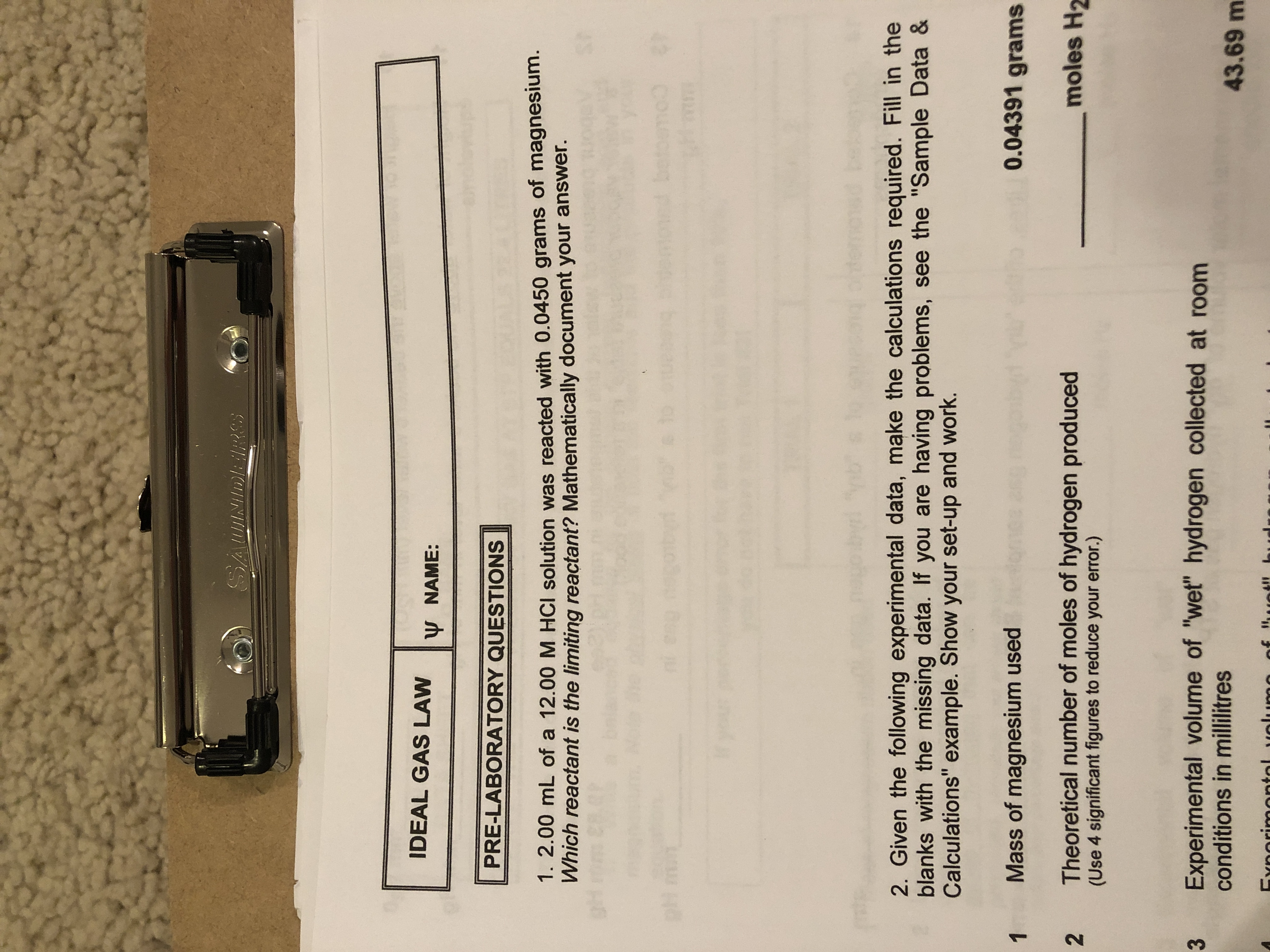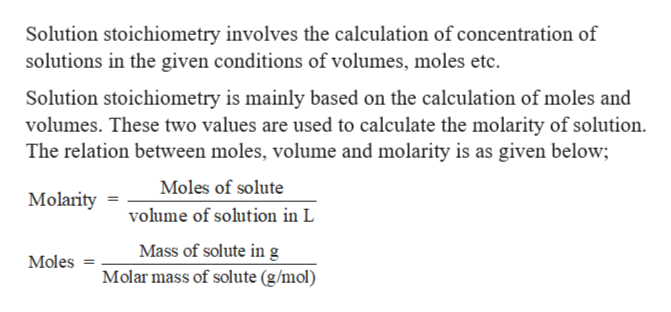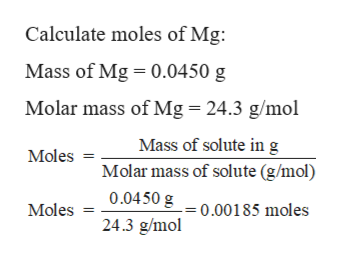# SAWNDERSIDEAL GAS LAWW NAME:PRE-LABORATORY QUESTIONS12.00 mL of a 12.00 M HCI solution was reacted with 0.0450 grams of magnesium.Which reactant is the limiting reactant? Mathematically document your answer.S70 81eh2. Given the following experimental data, make the calculations required. Fill in theblanks with the missing data. If you are having problems, see the "Sample Data &Calculations" example. Show your set-up and work.0.04391 gramsMass of magnesium used1Theoretical number of moles of hydrogen produced(Use 4 significant figures to reduce your error.)moles H22Experimental volume of "wet" hydrogen collected at roomconditions in millilitres343.69 mCvnorinoontaIvoluaf iI,

Question
1 views

Question 1help_outlineImage TranscriptioncloseSAWNDERS IDEAL GAS LAW W NAME: PRE-LABORATORY QUESTIONS 12.00 mL of a 12.00 M HCI solution was reacted with 0.0450 grams of magnesium. Which reactant is the limiting reactant? Mathematically document your answer. S7 0 81 e h 2. Given the following experimental data, make the calculations required. Fill in the blanks with the missing data. If you are having problems, see the "Sample Data & Calculations" example. Show your set-up and work. 0.04391 grams Mass of magnesium used 1 Theoretical number of moles of hydrogen produced (Use 4 significant figures to reduce your error.) moles H2 2 Experimental volume of "wet" hydrogen collected at room conditions in millilitres 3 43.69 m CvnorinoontaIvolu af iI, fullscreen
check_circle

Step 1help_outlineImage TranscriptioncloseSolution stoichiometry involves the calculation of concentration of solutions in the given conditions of volumes, moles etc. Solution stoichiometry is mainly based on the calculation of moles and volumes. These two values are used to calculate the molarity of solution The relation between moles, volume and molarity is as given below; Moles of solute Molarity volume of solution in L Mass of solute ing Molar mass of solute (g/mol) Moles fullscreen
Step 2
Step 3help_outlineImage TranscriptioncloseCalculate moles of Mg Mass of Mg 0.0450 g Molar mass of Mg 24.3 g/mol Mass of solute in g Moles Molar mass of solute (g/mol) 0.0450 g = 0.001 85 moles Moles 24.3 g/mol fullscreen

### Want to see the full answer?

See Solution

#### Want to see this answer and more?

Solutions are written by subject experts who are available 24/7. Questions are typically answered within 1 hour.*

See Solution
*Response times may vary by subject and question.
Tagged in
ScienceChemistry

### Other